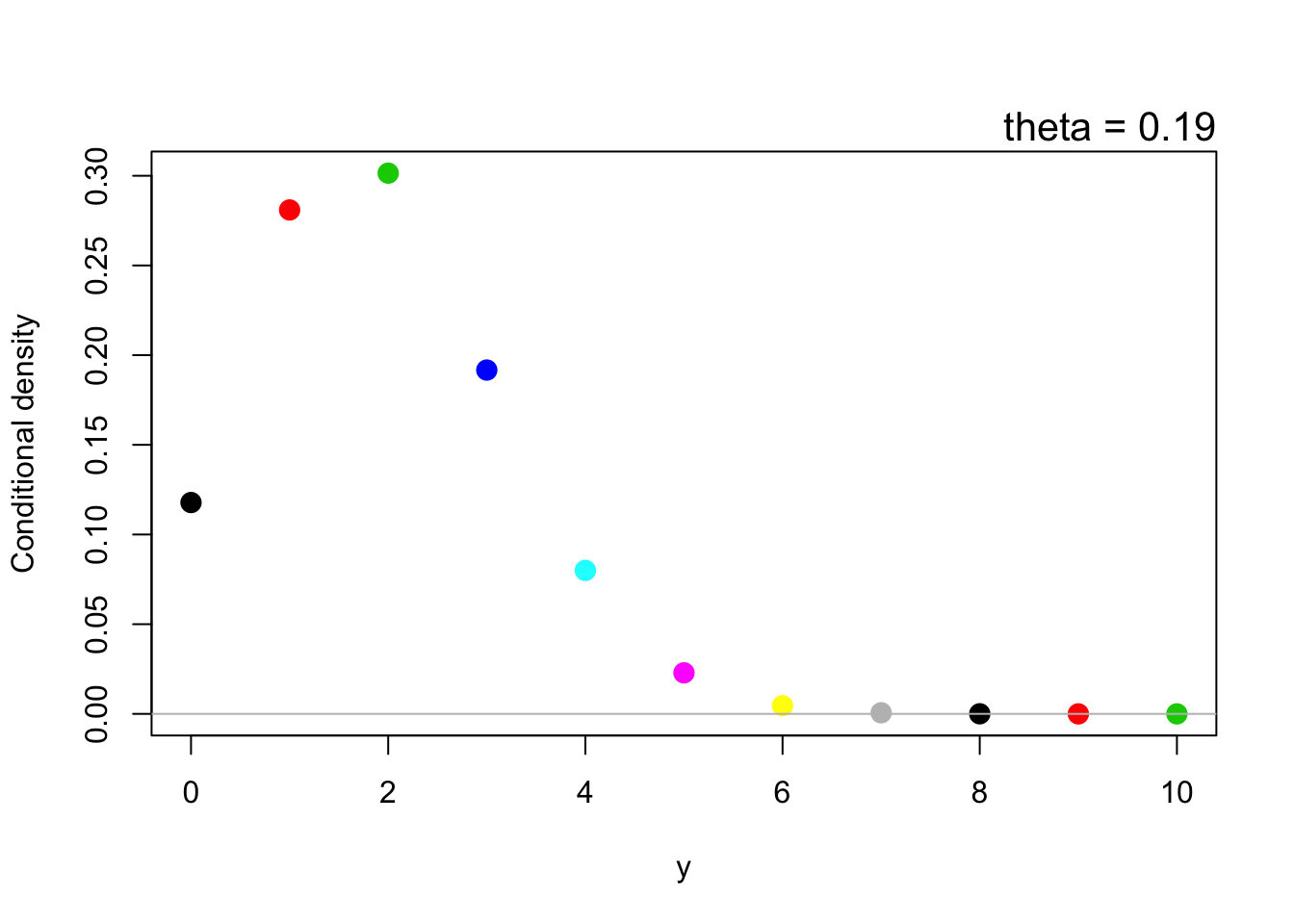## The model

We assume that the data have a binomial distribution with a probability parameter $$\theta$$, and we will observe $$N=10$$ binomial trials: $y \sim \mbox{Binomial}(N,\theta)$ which implies that $p(y\mid\theta) = \binom{N}{y}\theta^y(1-\theta)^{N-y}$

This function tells us the probability of various outcomes, assuming we knew the true $$\theta$$; for instance, the figure below shows the probability of each possible outcome from $$y=0$$ to $$y=10$$, assuming that $$\theta=0.19$$:Later on, it will be helpful to imagine that this is one of several possible sets of probabilities for $$y$$, embedded in a bivarate space with $$y$$ on one axis and $$\theta$$ on the other. The following 3D plot shows this (try rotating and zooming!):

Every value of $$\theta$$ corresponds to a new set of probabilities for the outcomes. Low values of $$\theta$$ will yield higher probabilities for smaller numbers of successes, while high values of $$\theta$$ will yield higher probabilities for the larger numbers of successes. We can visualize this with the following video:
Of interest to us is how to make an inference about $$\theta$$ after we observe $$y$$ successes out of $$N=10$$ trials.
Every Bayesian analysis begins with Bayes’ theorem. Since joint probability will be important to us, it will be helpful to think of Bayes theorem as a direct consequence of the definition of conditional probability: $p(\theta\mid y) = \frac{p(\theta, y)}{p(y)}.$ This is simply the definition of conditional probability. It implies that $p(\theta\mid y)p(y) = p(\theta, y) = p(y \mid \theta)p(\theta)$ which, of course, implies that $p(\theta\mid y) = \frac{p(y \mid \theta)p(\theta)}{p(y)}$ This is called Bayes’ theorem. We begin with a “prior” distribution $$p(\theta)$$ that quantifies a “reasonable belief” about $$\theta$$ (in some sense) before the data, and then arrive at a “posterior” distribution $$p(\theta\mid y)$$ that quantifies the “reasonable believe” we have about $$\theta$$ after observing the data.
For demonstration, I will use the following prior distribution for $$\theta$$: